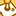# QlikView App Dev

Discussion Board for collaboration related to QlikView App Development.

Announcements
Skip the ticket, Chat with Qlik Support instead for instant assistance.
cancel
Showing results for
Did you mean:Contributor II

## equivalent for excel SLOPE function

Hi,

Is there any equivalent for Excel SLOPE function.

https://support.office.com/en-us/article/SLOPE-function-11fb8f97-3117-4813-98aa-61d7e01276b9

Regards

Łukasz

1 Solution

Accepted SolutionsMVP

Month, Value

1,94%

2,99%

3,99%

];

In the frontend, create a text box with expression

=LINEST_M( Value, Month)

It returns 0.025, i.e. 2.5% which seems reasonable to me.

4 RepliesMVP

Look into the linest_m function:

linest_m ([{set_expression}][ distinct ] [total [<fld {,fld}>] ]y-expression, x-expression[, y0 [, x0 ]])

returns the aggregated m value (slope) of a linear regression defined by the equation y=mx+b for a series of coordinates represented by paired numbers in x-expression and y-expression iterated over the chart dimension(s). Text values, null values and missing values in any or both pieces of a data-pair will result in the entire data-pair to be disregarded.

An optional valye y0 may be stated forcing the regression line to pass through the y-axis at a given point. By stating both y0 and x0 it is possible to force the regression line to pass through a single fixed coordinate.

Unless both y0 and x0 are stated, the function requires at least two valid data-pairs to calculate. If y0 and x0 are stated, a single data pair will do.

This function has the same limitations for nested aggregation as the avg([{set_expression}] [ distinct ] [ total [<fld { , fld } >]] expression) function. The linest_m function supports Set Analysis and the total qualifier in the same way as the avg([{set_expression}] [ distinct ] [ total [<fld { , fld } >]] expression) function.

Examples:

linest_m( Y, X )

linest_m( A/B, X*Y/3 )

linest_m( total Y, X )

linest_m( total <Z> Y, X)

linest_m( Y, X, 0 )

linest_m( Y, X, 1, 1 )Contributor II
Author

Thank you for the information and examples.
I have a question  how to write an expression.

I have a measure "hits%", and I wanna determine the slope for 3 months.

For Example I have values 94.% 99% 99%, and months numbered 1,2,3.

In excel I get slope = 3%MVP

Month, Value

1,94%

2,99%

3,99%

];

In the frontend, create a text box with expression

=LINEST_M( Value, Month)

It returns 0.025, i.e. 2.5% which seems reasonable to me.Contributor II
Author

I have one small problem.

I have a lot of "values" for various periods.

I want to count linest_m for 3 months back

i try to use

LINEST_M( sum({<Month={'>=\$(=only(Month)-2)<=\$(=only(Month))'}>}hits) , Month)

or

LINEST_M(  sum(aggr(rangesum(above(total sum({<Month=>}hits),0,3)),Month)), Month)

but it's doesn't workCommunity Browser